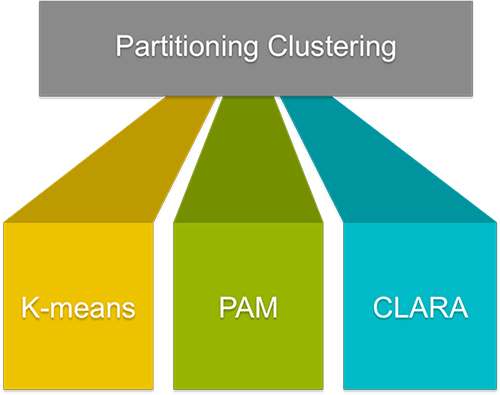Want to share your content on R-bloggers? click here if you have a blog, or here if you don't.

In this first volume of symplyR, we are excited to share our Practical Guides to Partioning Clustering.The course materials contain 3 chapters organized as follow:

K-Means Clustering Essentials

Contents:

• K-means basic ideas
• K-means algorithm
• Computing k-means clustering in R
• Data
• Required R packages and functions: stats::kmeans()
• Estimating the optimal number of clusters: factoextra::fviz_nbclust()
• Computing k-means clustering
• Accessing to the results of kmeans() function
• Visualizing k-means clusters: factoextra::fviz_cluster()
• Alternative to k-means clustering

K-Medoids Essentials: PAM clustering

Contents:

• PAM concept
• PAM algorithm
• Computing PAM in R
• Data
• Required R packages and functions: cluster::pam() or fpc::pamk()
• Estimating the optimal number of clusters: factoextra::fviz_nbclust()
• Computing PAM clustering
• Accessing to the results of the pam() function
• Visualizing PAM clusters: factoextra::fviz_cluster()

CLARA – Clustering Large Applications

Contents:

• CLARA concept
• CLARA Algorithm
• Computing CLARA in R
• Data format and preparation
• Required R packages and functions: cluster::clara()
• Estimating the optimal number of clusters: factoextra::fviz_nbclust()
• Computing CLARA
• Visualizing CLARA clusters: factoextra::fviz_cluster()

Example of plots: